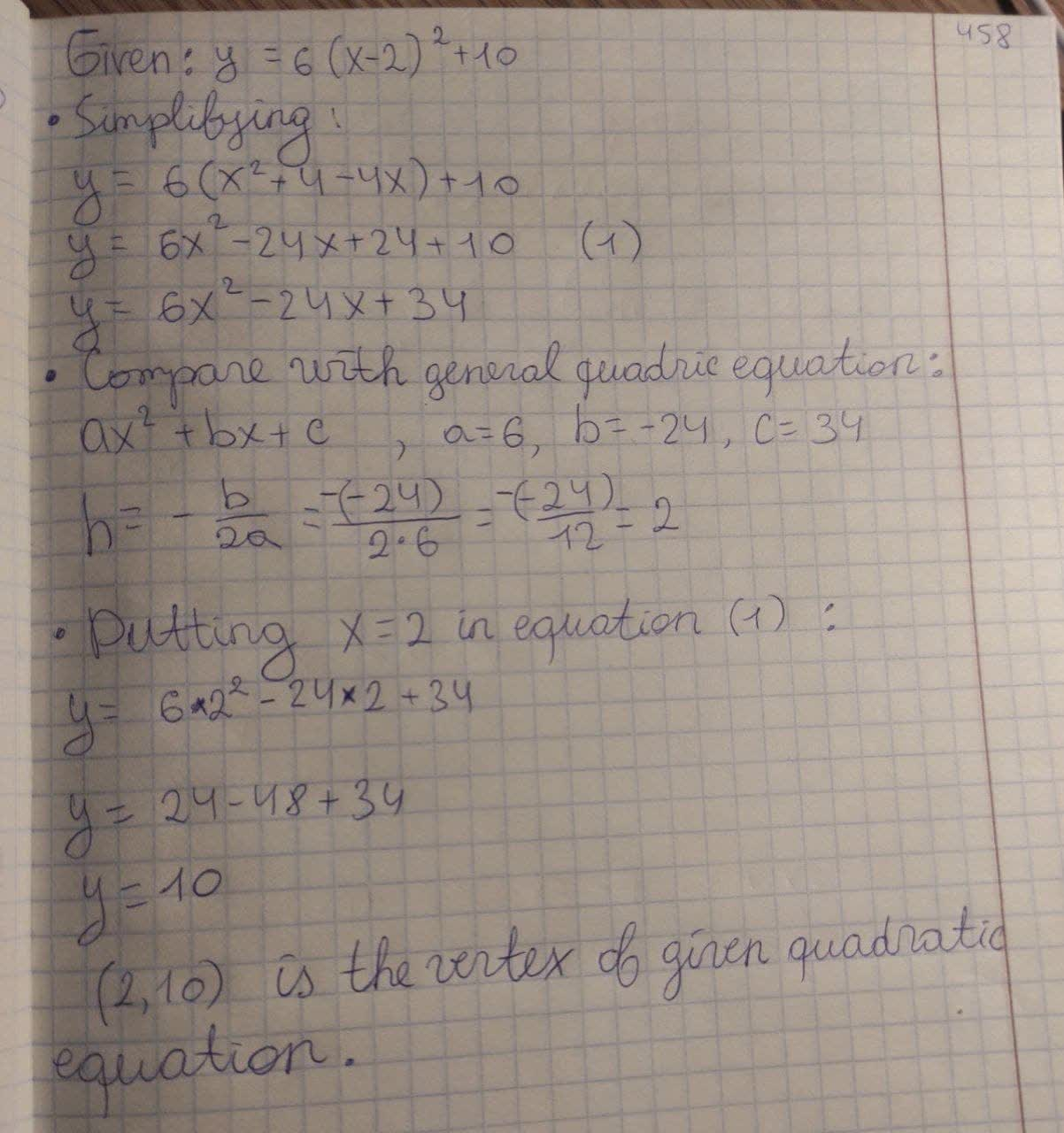What is the vertex of the quadratic y = 6 ( x − 2 ) 2 + 10?Mylo O'Moore 2021-02-22 Answered

What is the vertex of the quadratic $$y = 6 ( x − 2 )^2 + 10$$?

• Questions are typically answered in as fast as 30 minutes

Solve your problem for the price of one coffee

• Math expert for every subject
• Pay only if we can solve itLatisha Oneil
$$\displaystyle{y}={6}{\left({x}-{2}\right)}^{{2}}+{10}$$
On simplifying,
$$\displaystyle{y}={6}{\left({x}^{{2}}+{4}-{4}{x}\right)}+{10}$$
$$\displaystyle{y}={6}{x}^{{2}}-{24}{x}+{24}+{10}$$ (1)
$$\displaystyle{y}={6}{x}^{{2}}-{24}{x}+{34}$$
Now, this equation compare with general quadratic equation,
$$\displaystyle{a}{x}^{{2}}+{b}{x}+{c}$$
a = 6 , b = -24 , c = 34
$$\displaystyle{h}=-\frac{{b}}{{2}}{a}$$
$$\displaystyle=-\frac{{-{24}}}{{{2}\cdot{6}}}$$
= 2
Now putting x= 2 in equation (i), then we get
$$\displaystyle{y}={6}\cdot{2}^{{2}}-{24}\cdot{2}+{34}$$
y = 24 - 48 + 34
y = 10
(2, 10) is the vertex of given quadratic equation.